# iFit: Neutron Scattering procedures

1. Neutron scattering Dynamic structure factor
2. Isotropic dynamic structure factors S(|q|,w) in liquids, powders, gas, polymers and other amorphous materials
2. Neutron scattering cross sections for nuclear engineering
3. Neutron diffraction
4. Neutron Triple-Axis Spectrometers
5. Neutron Scattering Instruments with McStas

## Neutron scattering Dynamic structure factor

The iFit infrastructure comes with a set of dedicated objects and methods to read and analyze S(q,w) dynamic structure factors, aka scattering laws, in the case of neutron scattering [5,6].

The dynamic structure factor is defined from the double differential scattering cross section per unit solid angle and final neutron energy as [5,6] (here for a monoatomic material):

d2σ/dΩdEf = Nσb /4π kf/ki S(q,w)

where N σb kf and ki are the number of scattering units, their bound scattering cross section , the final and initial neutron wave-vector, respectively.

You may as well generate 4D S(q,w) models using e.g. the sqw_phonons and other specialized models. Then you can compute the 2D powder average, as shown below.

It is also possible to generate 2D S(q,w) models from analytical expressions, or a density of states using the so-called incoherent Gaussian approximation.

The iFit objects which are handled below are:
• the generic iData data set
• the S(q,w) 2D iData_Sqw2D flavour, data set
• the S(alpha,beta) 2D iData_Sab for nuclear data sets (thermal scattering laws)
• the g(w) 1D iData_vDOS representing a vibrational density of states
• the generic iFunc model
• the S(q,w) 2D model iFunc_Sqw2D for e.g. liquids and powders
• the S(q,w) 4D model iFunc_Sqw4D from e.g. phonons and spin-waves in single-crystals

In this section, we describe how one can load existing data sets, or generate ones.

A 2D S(|q|,w) consists in a momentum axis q (wavevector, usually in Angs-1), an energy axis w (usually in meV), and a dynamic structure factor 2D matrix S(q,w).

#### From an experiment, processed data

Such data sets can be measured on neutron scattering spectrometers (time-of-flight or triple-axis). Once the raw data has been reduced, using e.g. LAMP at the ILL:

1. apply scripts normalise, vnorm, corr_tof, t2e, and finally sqw_rebin) ;
2. you get a S(q,w) data set as a workspace ;
3. export it into a HDF/NeXus file or as text (write_lamp, format='hdf').

Similar data sets can be measured using X-ray spectrometers.

The file can then be read by iFit, using any of

• Sqw=iData('file')

which can read HDF, (for instance HDF/NeXus from Mantid) text, NetCDF, and many other formats (see Loaders for a full description).

It is then advisable to convert the generic iData object into a specific 2D S(q,w) flavour iData_Sqw2D, so that the methods below are applicable. This can be done automatically when importing a file, as shown in the 2nd example

• Sqw=iData_Sqw2D(Sqw)
• Sqw=iData_Sqw2D('file')
• Sqw=iData_Sqw2D('SQW_coh_lGe.nc')

Common formats that can be read include McStas Isotropic Sqw, ISIS SPE and SQW, ILL ToF experiments, ENDF (see below).

NOTE: it is required that the 2D data set axes allow to identify their type, e.g. the labels match 'angle','time','momentum','energy', ...

#### From an experiment, raw data

Direct experimental data can be automatically converted to S(q,w) from any of (e.g. from ToF experiment):

• S(φ,channel) where φ is the radial scattering angle, and channel is the channel id (integer, step=1). This conversion requires to locate the experimental neutron wavelength (searched as 'lambda' or 'wavelength' in the data set), the sample to detector distance (searched as 'distance', as a scalar for radial geometry, or a vector for more complex geometries), and the time channel width (searched as 'ChannelWidth', scalar or vector). The time channel width can be given in [s] (preferred), [ms], or [us].
• S(φ,t) where φ is the radial scattering angle, and t is the detection time counted from the sample location. This conversion requires to locate the experimental neutron wavelength (searched as 'lambda' or 'wavelength' in the data set), the sample to detector distance (searched as 'distance', as a scalar for radial geometry, or a vector for more complex geometries). The time can be given in [s] (preferred), [ms], or [us].
• S(φ,w) where φ is the radial scattering angle and w is the energy transfer (in [meV]). This conversion requires to locate the experimental neutron wavelength (searched as 'lambda' or 'wavelength' in the data set).
The conversion takes place when calling iData_Sqw2D:
• Sqw=iData('file') % it may be a (phi, ToF) data set
• Sqw = iData_Sqw2D(Sqw); % when needed the data is converted to (q,w) space
NOTE: it is required that the 2D data set axes allow to identify their type, e.g. the variable names or labels match 'angle','time','momentum','energy', ...

#### From Matlab variables

If you managed to get a matrix S and two vectors q,w as Matlab variables, create an S(q,w) data set with:
• Sqw=iData(q,w,S)
• Sqw=iData_Sqw2D(Sqw)
NOTE: it is required that the 2D data set axes allow to identify their type, e.g. the labels match 'angle','time','momentum','energy', ...

#### From a Model (iFunc)

If S is a 2D iFunc Model (analytical, such as obtained from the sqw_phonons), then simply evaluate the model into an iData object, with default Model parameters [], or specified ones. Axes must be specified as momentum and energy values in [Angs-1] and [meV] resp.

• Sqw=iData(S, parameters, q, w)
• Sqw=iData_Sqw2D(Sqw)
It is also possible to obtain a 2D data set from a 4D iFunc_Sqw4D model by performing e.g. a powder average (see powder method below), and then an evaluation.
• model4d = sqw_cubic_monoatomic
• model4d = sqw_phonons('defaults') % using ASE/PhonoPy and DFT calculator
• model4d = sqw_spinw('defaults') % PSI SpinW S. Toth
In order to apply the powder method, the model must be converted to an iFunc_Sqw4D flavour (which may not be needed if already generated as such)
• model4d = iFunc_Sqw4D(model4d)
Then apply the powder average:
• model2d = powder(model4d)
• Sqw = iData(model2d, parameters, q, w, ...)

#### Shaping the Sqw data set

The data set may extend on both negative and positive energy transfers, or only on one side.

If it has only positive or negative energy values, then create the other side using the symmetrize method, as detailed below.

You may as well apply the following iData operators:
• resizechange binning in q,w, fast rebin.
• reshape: change the dimensions of the Sqw data set, the number of elements must not change.
• fill: replaces missing data (NaN's, gaps) by interpolating
• smooth: smooth the data set
• meshgrid: creates a square 2D distribution, using interpolation if necessary.
• powder: converts a 4D S(hkl,w) model into a 2D S(q,w) one (see below).
• xlim: the 'x' axis is the momemtum/wavevector 'Q' e.g. in [Angs-1]. It corresponds with the axis of rank 2, in the way Matlab switches xy axes for plots. To change the Q axis use xlim(Sqw, [min max]).
• ylim: the 'y' axis is the Energy transfer 'w' e.g. in [meV]. It corresponds with the axis of rank 1, in the way Matlab switches xy axes for plots. To change the w axis use ylim(Sqw, [min max]).
and you should of course plot it, e.g. with:
• plot(log(Sqw))

### Isotropic dynamic structure factors S(|q|,w) in liquids, powders, gas, polymers and other amorphous materials

The following procedures apply to 2D S(q,w), i.e. those that are obtained by averaging the S(q,w) over |q| in isotropic density materials.
In the following, S*(q,w) is a symmetric (classical) dynamic structure factor, whereas S(q,w) denotes the non-symmetric (quantum) dynamic structure factor which contains the population factor (e.g. Bose for phonons). See below for more details on this.

All routines below call the iData_Sqw2D conversion/check, which makes sure the S(q,w) data satisfies the format definition. This routine also makes, when needed, the conversion from an experimental to the S(q,w) format (change of axes, taking into account the Jacobian).

Conventions:
• w = Ei-Ef = energy lost by the neutron in meV.
• w > 0, neutron looses energy, material/sample gains energy, w can not be higher than the incident neutron energy (Stokes)
• w < 0, neutron gains energy, material/sample looses energy (anti-Stokes)
• q = Ki-Kf is the momentum exchange (or wavevector) in Angs-1.
If the 'positive' energy transfer side does not corresponds with the neutron loss side (sample gains energy from the neutron), then you should revert the energy axis with e.g.:
• setaxis(Sqw, 1, -Sqw{1}) or equally Sqw{1} = - Sqw{1};
All routines assume the wavevector 'q' is given in [Angs-1] and the energy is given in [meV].

1 meV = 241.8 GHz = 11.604 K = 0.0965 kJ/mol = 8.0657 cm-1
1 Angs-1 = 10 nm-1

 Procedure Input Output Description Sqw=load(iData,file) Sqw=iData(file) Sqw=iData_Sqw2D(file) file iData or iData_Sqw2D Import any 2D S(q,w) as generated by e.g. LAMP, Mantid and nMoldyn/MDANSE. Possibly convert from experimental data S(φ,channel), S(φ,t) or S(φ,w). Sqw=iData_Sqw2D(iData(q,w,S)) [q,w,S] iData_Sqw2D Import data set S as a function of axes q and w. Sqw=iData_Sqw2D(sab) iData_Sab S*(alpha,beta) iData_Sqw2D Import an S(alpha,beta) symmetrize(sqw2d) iData_Sqw2D S*(|q|,w>0) S*(|q|,w)=S*(|q|,-w)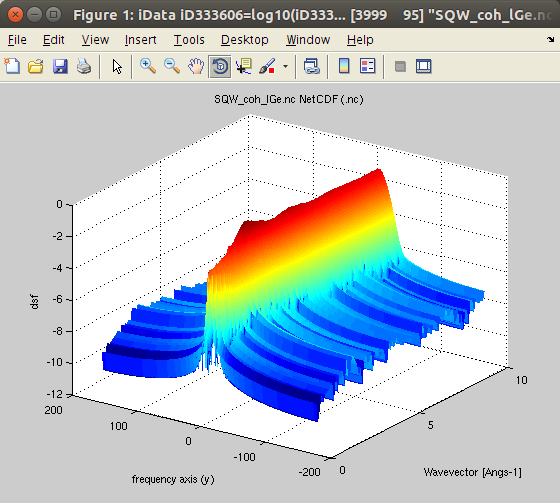Extend the S*(|q|,w) in both energy sides. The initial data set should better be 'classical'. Bosify(sqw2d,T) iData_Sqw2D S*(|q|,w) S(|q|,w)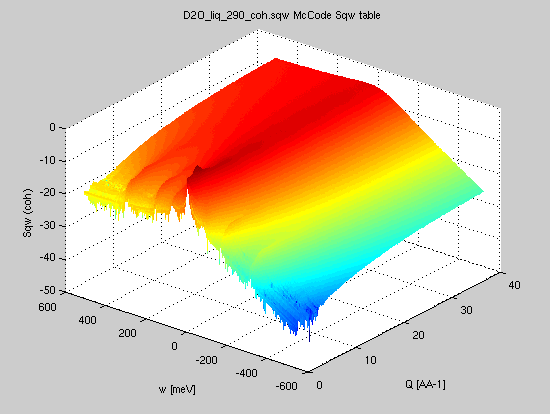Convert a symmetric scattering law (aka classical, for instance from Molecular Dynamics) into a 'quantum' scattering law (non symmetric, for instance from neutron and X-ray inelastic scattering experiment) iData_Sqw2D(sqw2d) iData_Sqw2D('file') S(|q|,w) or S*(|q|,w) S(|q|,w) or S*(|q|,w) Check the S(q,w) data set. Possibly convert from experimental data S(φ,channel), S(φ,t) or S(φ,w). deBosify(sqw2d,T) iData_Sqw2D S(|q|,w) S*(|q|,w) Convert a 'quantum' scattering law (non symmetric, for instance from neutron and X-ray inelastic scattering experiment) into a symmetric scattering law (aka classical, for instance from Molecular Dynamics) trapz(sqw2d) structure_factor(sqw2d) iData_Sqw2D S(|q|,w) S(|q|)Compute the static structure factor. The S(q) is about the same when computed from the 'classical' and the 'quantum' scattering law. dynamic_range(sqw2d,Ei) iData_Sqw2D S(|q|,w) or S*(|q|,w) measurable S(|q|,w) for Ei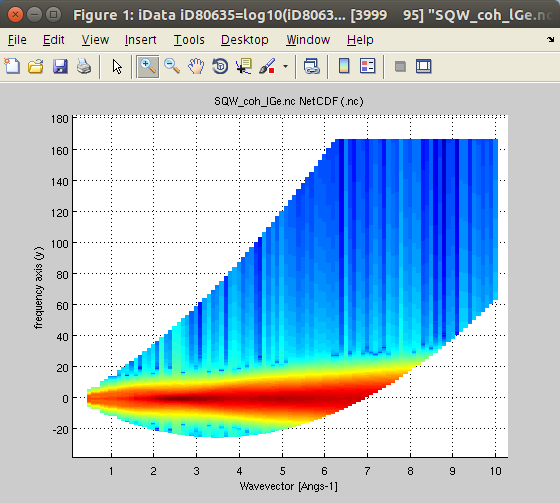Restrict the dynamic structure factor to the measurable one for an incoming energy Ei [meV]. The detection angular range can be set as 3rd argument. moments(sqw2d,M) iData_Sqw2D S(|q|,w) or S*(|q|,w) S(q), Er, characteristic frequencies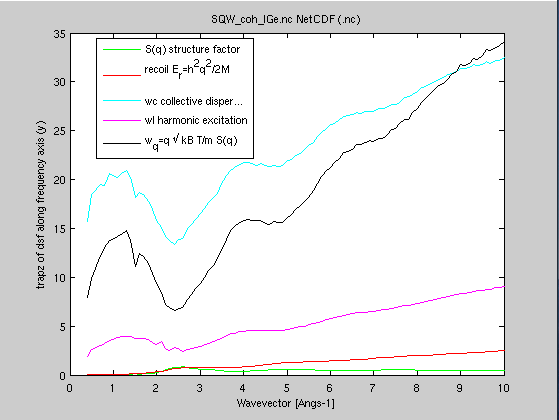Compute the energy moments, and provide some of the characteristic frequencies (recoil, isothermal, longitudinal/harmonic, mean energy transfer) dos(sqw2d) iData_Sqw2D S(|q|,w) or S*(|q|,w)or S(q,w) g(w) Compute the vibrational density of states (DOS) using Bredov or Carpenter methods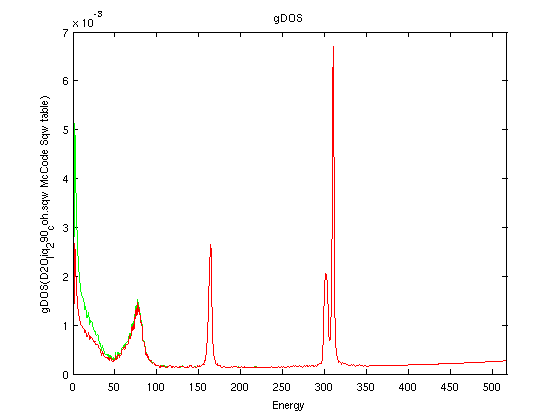scattering_cross_section(sqw2d,Ei) iData_Sqw2D S(|q|,w) ∫∫S(q,w)q dq dw /2Ki2Compute the total thermal neutron scattering cross section which is the integral of S(q,w) over the dynamic range vs. incident neutron energy. Sab(sqw2d, M) iData_Sqw2D S*(|q|,w) iData_Sab S*(alpha,beta)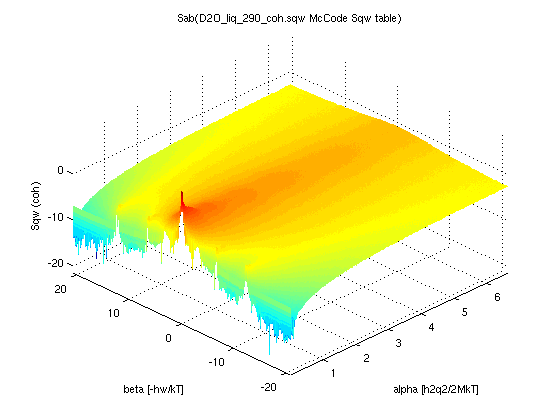Convert a dynamic structure factor to its unit-less representation S(α,β) powder(sqw4d model) iFunc_Sqw4D S(hkl,w) 4D model iFunc_Sqw2D model S(|q|,w)Convert a 4D S(hkl,w) model into a powder averaged S(|q|,w) model. This can be used e.g. after calling sqw_phonons and other 4D S(q,w). max(Sqw4d model) iFunc_Sqw4D S(hkl,w) 4D model energy maximum Computes the maximum energy of modes in a 4D S(h,k,l,w) model. Also returns a fast evaluation of the vDOS. powder(sqw4d) iData_Sqw4D S(hkl,w) 4D iData_Sqw2D S(|q|,w) Convert a 4D S(h,k,l,w) data set into S(|q|,w). band_structure(sqw4d model) iFunc_Sqw4D S(hkl,w) 4D dispersion curves Compute the dispersion curves and neutron intensity along the main crystal axes. publish(sqw4d model) iFunc_Sqw4D S(hkl,w) 4D HTML page Write a full document about a 4D S(h,k,l,w) model. thermochemistry(sqw4d or g, T) iFunc_Sqw4D S(hkl,w) 4D or iData_vDOS g(w) U,F,S,Cv Compute the energy, entropy, heat capacity, and also returns the DOS. iData_vDOS(g) iData g(w) 1D iData_vDOS Convert a 1D data set assumed to be a vDOS incoherent(g) iData_vDOS g(w) iData_Sqw2D array Compute the incoherent S(q,w) in the Gaussian/multi-phonon approximation. multi_phonons(g) iData_vDOS g(w) iData_vDOS 1D array Compute the effective neutron weighted density of states separate terms, which contain multi-phonon terms. gdos(g) iData_vDOS g(w) iData_vDOS Compute the effective neutron weighted density of states sum, which contains multi-phonon terms.

#### Dynamic structure factor from Molecular Dynamics (MD)

In the following, we demonstrate the different treatment procedures, starting from an example data set obtained from a molecular dynamics simulation.

We import the data, obtained from an ab-initio Molecular Dynamics (AIMD) with VASP, for 200 Ge atoms (GGA-PW91 potential) at T=1350K, from . The data set is part of iFit, as well as in McStas. You can also try liquid Rubidium from here  and heavy water from here . This is the coherent symmetric scattering law:
• Sqw=iData_Sqw2D('SQW_coh_lGe.nc')
Molecular Dynamics trajectories are usually computed in the NVE micro-canonical ensemble. The integrator used along the trajectory is the Verlet, which is a central difference, energy conserving integrator. As a consequence, the time evolution is symmetric (i.e. trajectories can be read in positive or negative time steps without changing the system behaviour). This implies that the derived intermediate scattering function F(q,t) is real, symmetric in time, and as a consequence S(q,w) is also real, symmetric in energy. We denote this scattering law S* to label it as symmetric.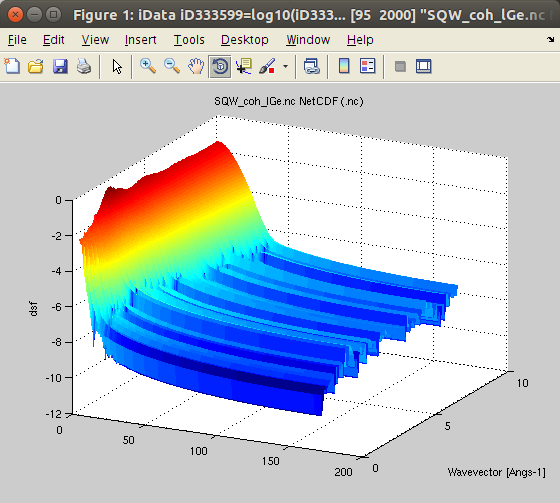It is often named 'classical'.

We plot the scattering law S*:
• plot(log10(Sqw), 'view3');
Then we need to symmetrize the data set, as it comes defined only in w>=0. This procedure applies to a 'classical' symmetric S*(q,w). If the scattering law you have already extends on both energy sides, you can skip this step (e.g. nMoldyn4/MDANSE).
• Sqw2=symmetrize(Sqw)
Then we can plot the symmetrized scattering law, which extends equally in both w<0 and w>0.• plot(log10(Sqw2), 'view3');
We can check here that:
S*(q,-w) = S*(q,w).

Note: if the initial S(q,w) is already normalised so that lim S(q → Inf) = 1 , then the resulting symmetrized data set should be divided by a factor 2.

##### From classical to quantum scattering law (quantum correction, detailed balance, Bose factor)
The next step is to get the temperature in.

A warning is in place here
. There is only one S(q,w), but there are infinite ways to derive a symmetrized S*(q,w). So, having obtained S* from Molecular Dynamics, we have to choose how to 'desymmetrize' it in order to obey the so-called 'detailed balance' accounting for the population of vibrational modes [5,6]:

S(q,w)  = exp(hw/kT) S(q,-w)

This step is performed by the Bosify procedure, which can use 3 different 'quantum corrections' to go from a classical/symmetric S*(q,w) data set to a 'real/quantum' scattering law.

S(q,w)  = Q(w) S*(q,w) with S*=classical limit

The semi-classical correction, Q, aka 'quantum' correction factor,  can be selected as [1,2,34]:

 Q = exp(hw/kT/2) 'Schofield' or 'Boltzmann'  Q = hw_kT./(1-exp(-hw/kT)) 'harmonic'  or 'Bader'  Q = 2./(1+exp(-hw/kT)) 'standard'  or 'Frommhold' . This is the default.

where hw is the energy (in meV), and k is the Boltzmann constant.

Even though the 'Boltzmann' correction is the most natural correction, as it directly derives from the detailed balance, it is not recommended as it leads to a divergence of the S(q,w) for large energies e.g. above few 100 meV. The 'harmonic' correction is weaker but does not fully avoid the divergence at large energies. The 'standard' correction is probably the best when dealing with large neutron energies. In the 'classical' limit where hw << kT (and kT ~ 30 meV a 300K), all corrections are equivalent. All these corrections satisfy the detailed balance equally.

So, from the symmetric 'classical' S* we can now determine the 'true' S(q,w) scattering law, at a given temperature, using the 'standard' quantum correction.
• SqwT = Bosify(Sqw2, 1250)
• plot(log10(SqwT));When not given, the temperature will be searched in the Sqw data set. A 3rd argument allows to specify the quantum correction to apply ('standard' is default). Of course, in this example, for such a high temperature, the up- and down-scattering are about the same (the quantum correction remains close to 1). For materials at low temperatures, the two sides are highly disymmetric.

The deBosify procedure performs the opposite operation, that is compute a symmetric S*(q,w) from e.g. a 'quantum' experimental S(q,w). It corresponds with Bosify(Sqw, -T).

##### Dynamic range accessible for a given neutron incident energy, structure factor, inelasticity correction
The dynamic range is computed by applying the momentum and energy conservation rules to the S(q,w):

Ef = Ei - w is positive
cos(θ) = (Ki2 + Kf2 - q2) / (2 Ki.Kf)  is within [-1:1]

where Ei and Ef are the incident and final neutron energies, θ is the scattering angle, Ki and Kf are the incident and final neutron wavevectors, defined as:

Ei = 2.0721*Ki2 = 81.8042/λ2 with Ki in [Angs-1] and λ in [Angs]

We can determine the dynamic range accessible to neutrons with a given incident energy, say Ei=25 meV.
• Sqw25 = dynamic_range(SqwT, 25)
• plot(log10(Sqw25));
The integrated scattering cross section is the integral of the dynamic range, for a given incident neutron energy (see below).The structure factor S(q) is the integral of S(q,w) over the energy. We use the usual 'trapz' integrator, or structure_factor which does the same.

S(q) = ∫S(q,w) dq

In addition, the structure factor should converge to 1 at high momentum values:

lim S(q → Inf) = 1

We then recommend to plot the structure factor, and possibly adapt the S(q,w) value so that the high wavevector S(q) limit should tend to 1. This is simply accomplished by dividing the dynamic structure factor S(q,w) by the structure factor limit S(q → Inf).

It is here possible to compare the structure factor as measured with Ei=25 meV (i.e. restricted to the corresponding dynamic range) to the ideal one. The ratio is called the "inelasticity correction". Also, on a real experiment, the integration over momentum and angle is not identical, raising additional corrections.
• plot(structure_factor([SqwT, Sqw25]));
#####Characteristic frequencies (moments)
From the dynamic structure factor, one can compute the energy moments, which provide some of the characteristic frequencies [5,6,10], specifying the molar weight m of the scattering units (here 72.64 g/mol for Ge).

Using the 'quantum' scattering law S(q,w), we can define n-th order frequency moment as:

<wn S> = ∫ wn S(q,w) dw

and then derive:
• S(q) = ∫S(q,w) dq = <S> is the 0-th frequency moment
• Er(q) = h2q2/2M = <w S> is the recoil energy, 1st frequency moment
• Wc(q) = √[ 2kT*Er(q)/S(q) ] is the collective/isothermal dispersion
• Wl(q) =√[ <w3S>/Er(q) ] is the harmonic/longitudinal excitation
• Wq(q) = 2q √[ kT / m.S(q) ] is the mean energy transfer, or half width from normalized 2nd frequency moment
These frequencies are computed with:
• moments=moments(SqwT, 72.64)
• plot(moments(1:5))
The moments can equally be computed from a 'classical' symmetric S*(q,w) and a 'quantum' experimental S(q,w).
##### The density of states (aka phonon, vibrational or frequency spectrum)
The scattering law, from its definition [5,6], is a correlation function of the density operator, which itself depends on the position of the particles.
The vibrational density of states is defined as the velocity auto-correlation function (VACF) of the particles. It is not a function of the positions. So, in principle, it is NOT possible to compute the vibrational density of states from the scattering law.

However, a few attempts have been made to estimate the density of states from e.g. a neutron scattering time-of-flight experiment (such as done on IN4, IN5 and IN6 at the ILL). The concept of the generalised density of states has been defined as [Carpenter/Bellissent 12,13,15]:

gDOS(q,w) = S(q,w) w  /q^2/[1 + n(w)] ~ S(q,w) w2/q2

which is valid in the case of an incoherent scatterer. Here, n(w) is the Bose factor. Then, the energy dependent density of states is the low-wavevector limit of the generalised density of states, which is a normalized quantity:

g(w) ~ gDOS(q → 0,w)

∫g(w) dw = 1

From an experimental S(q,w) data set, the g(w) is obtained from the intensity in the low angle detector bank vs. the energy transfer. As such, it should only be considered as an approximation of the vibrational density of states, especially for most materials which have coherent scattering. However, the Carpenter method is satisfactory for incoherent materials, and results from Molecular Dynamics where the q range reaches low q values. The hydrogenated materials and e.g Vanadium are good examples of materials which are mostly incoherent, and the approximation is then good. For other materials, great care should be taken.

The Bredov/Oskotskii method  is also valid for coherent scatterers, with better statistics:

gDOS(q,w) = w q S(q,w) e2W(q) /[Qmax4 - Qmin4]/(1+n(w))
gDOS(w) = ∫ gDOS(q,w) dq

where Qmin and Qmax are the minimal and maximal momentum values reachable in the dynamic range of the neutron spectrometer. The Carpenter and Bredov methods usually provide different results.

The syntax to compute the density of states is usually:
g = dos(sqw2d) % uses Bredov method by default
g = dos(sqw2d, 'Carpenter')
g = dos(s, 'method', method, 'T', T, 'DW', dw)
We import the heavy water NAMD simulation (3900 molecules, 290K, E. Pellegrini) coherent dynamic structure factor from here .
Then we can compute the generalised density of states with the dos procedure, from both the raw, and the symmetrized, 'Bosified' (with quantum correction) data set.
• SqwT = Bosify(symmetrize(Sqw), 290);
• plot(log(SqwT))
• g=[ dos(Sqw, 'Carpenter')) dos(SqwT, 'Carpenter')) ];
• plot(g); xlim([0 Inf]);It is also possible to evaluate the density of states from a 4D S(q,w) model, using:
g = dos(model4d)
Refer to the Models/Phonons page.
##### The powder averageWhen a 4D S(q,w) model is created, using e.g. the sqw_phonons and other specialized models, one  can compute the 2D powder average, which is the projection of the 4D data set onto the (|q|,w). space.

However, this projection must take care of the crystal structure. When the reciprocal lattice information is found in the object (matrix B=[a* b* c*]), the conversion from 'rlu' (e.g. 2pi/a units) to 'Angs-1' (cartesian) is automatically done (and this information is stored in the Model upon creation):

Qcart = B*Qrlu

The the average is obtained using e.g. the powder method
`	>> s=sqw_phonons([ ifitpath 'Data/POSCAR_Al'],'metal','EMT'); 	>> pow=powder(s); plot(log(pow));`
Here we have generated a 4D data set from the sqw_phonons iFunc Model, and then reduced it to 2D.
The same procedure can be applied on an evaluated iData object out of the model.

#### Dynamic structure factor from a neutron scattering experiment

The symmetrization step is not needed if you import experimental S(q,w) as measured on inelastic neutron scattering spectrometers such as IN5@ILL. This type of data set already 'contains' the Bose factor accounting for the population of modes.

Then the sequence of operations is first to load the data set, then apply the deBosify procedure, specifying the temperature if it is not defined in the data set. Then symmetrize can be applied to derive a symmetric data set which extends in both w>0and w<0. The data close to the elastic w=0 line, which can be measured in up- and down- scattering, is computed as the mean of both sides.

Then, the rest of the data treatment is the same as above.

#### Dynamic structure factor from a density of states

It is rather usual to derive a so-called density of states from a neutron or X-ray inelastic scattering experiment. Such quantity can also be estimated from e.g. ab-initio lattice dynamics (see our sqw_phonon model), perturbation theory, molecular dynamics (use e.g. MDANSE), ...

As defined above, the vDOS measures the density of vibrational states in [w, w+dw]. This quantity is central in the determination of thermochemistry quantities such as U, S, F, and Cv.

However, it can also be used to estimate the incoherent scattering law S(q,w) [Sjölander theory, Gaussian approximation] as well as a further estimate of the coherent scattering law [Sköld approximation].

In this way, we suggest that, from an inelastic neutron scattering experiment where the total intensity is measured, the data is corrected and reduced to extract the gDOS. Then, the incoherent approximation is used (see below), which can be subtracted from the total intensity to reveal the coherent part better and help in the 'background' subtraction.
##### Incoherent scattering law estimate: monoatomic material
The estimate of the incoherent scattering law is based on the so-called incoherent approximation, which states that any angle or momentum integrated quantity from both the incoherent and the coherent scattering processes are equal. This can be demonstrated in the case of a monoatomic cubic lattice material, and has been extended to other materials within a 20% difference in general.

In practice, this can be checked from any molecular dynamics modelling, computing first the theoretical density of states from the velocity auto-correlation function, as well as the incoherent and coherent scattering laws. Then, we can estimate from each S(q,w) the generalised density of states as defined above, and check that these two estimates are comparable.
As a consequence, the density of states can be estimated similarly from incoherent, coherent and even total scattering integrals .

The so-called Gaussian approximation (aka multi-phonons expansion) starts from the general expression of the scattering law. It is usual to write it:

d2σ/dΩdEf = kf/ki S(q,w)

where S contains the bound scattering cross section σ and the number of scatterers N (e.g. the mass or material density). In a very general expression, S contains the single excitation term, as well as further multi-excitation terms which are often omitted, assumed negligible.

In a poly-atomic material, the expression of S contains 'partial' terms as well as 'interference' (cross) terms:

S(q,w) = ∑ bib*j Sij(q,w)

where bi is the scattering length for atom 'i'. In practice we find that the 'partial' terms per atomic species contain a coherent (e.g. periodic features such as structure and vibrations) and an incoherent part (e.g. from disorder).

Sjölander  has derived an extensive theoretical development of the dynamic structure factor, including multi-excitations. However, this methodology can only be applied to the incoherent part. It may then seem odd to talk about multi-phonons in a incoherent scattering law which does not contain any phononic part. For a monoatomic isotropic material, it is possible to write:

S(Q,w) = N σinc /4π exp[ -hQ2/2m f(0) ] ∫ e-iwt exp[ hQ2/2m f(t) ] dt

where the function 'f' is computed from the density of states:

f(t) = ∫ eiwt g(w)/w (n(w)+1) dw

The second exponential term is then expanded in Taylor series, to draw:

S(Q,w) = N σinc /4π exp[ -h2Q2/2m f(0) ] ∑p 1/p! ∫ e-iwt [ h2Q2/2m f(t) ]p dt

hence the naming as 'phonon expansion'. This rather complex expression can be computed by introducing the time Fourier tranform of f(t)p

Tp(w) = ∫ e-iwt f(t)p dt

which satisfies:
T0(w) = δ(w)
T1(w) = g(w)/w (n(w)+1)
Tp   = T1 * Tp-1 with '*' being the convolution operator
These function T are normalized as:
|T1| = f(0) = ∫ T1(w) dw
|Tp| = f(0)p
from which we can estimate the Debye-Waller factor

W(Q)  = h2Q2/2m f(0)

The p=0 term is the Elastic Incoherent Structure Factor (mostly a Dirac peak with a decreasing Debye-Waller amplitude)

S(Q,w)[p=0] = (1/4π) e-2W(Q) δ(w)

The p=1 term is the 'one-phonon' response

S(Q,w)[p=1] = Nσinc/8πm Q2 e-2W(Q) g(w)/w (n(w)+1)

The following terms are obtained by iterative auto-convolution by the vDOS

S(q,w)[p]   = Nσinc/4π/!p e-2W(Q) [2W(q)]p Tp(w)

All this mechanics allows to estimate the S(q,w) as a sum of decreasing terms as 1/mp where m is the mass of the atom. So for most materials, the additional terms are negligible after e.g. p> 5. However, this methodology allows, only starting from the density of states, to estimate the incoherent scattering law. And it proves very efficient (fast to compute) and reasonably accurate.

This strategy is the one used in the NJOY/LEAPR module, known as "phonon expansion" to estimate the thermal scattering law in the incoherent approximation.

The use with iFit is simple. If one has a density of states g, the incoherent S(q,w) is obtained as:
• Sinc = incoherent(g)
If the atom mass (in [g/mol]) and Temperature (in [K]) need to be defined, the syntax becomes:
• Sinc = incoherent(g, 'm', m, 'T', T)
The final 2D Sinc(q,w) must be multiplied by the bound incoherent scattering cross section of the material.

As an example, we can get the density of states from an incoherent S(q,w) obtained by MD, then compute back the S(q,w) estimate. To estimate the DOS, we use the Carpenter method which is well suited to MD results containing low 'q' values such as here. The incoherent method is then used, specifying the momentum axis 'q' to use as the one from the MD data set, and the DW coefficient is set to a low value, as the MD does not simulate the DW attenuation along Q in S(q,w). Last, the temperature effect (Bose factor) is part of the incoherent estimate. To compare with the initial S(q,w) from MD which is 'classical' (symmetric), we 'deBosify' the incoherent estimate. The plot then restricts the energy range to only the positive one.
• Sinc0 = iData_Sqw2D('D2O_liq_290_inc.sqw.zip')
• gDOS = dos(Sinc0, 'Carpenter'); % well suited for MD results
• Sinc1 = incoherent(gDOS, 'DW', 0.0001,'q',Sinc{2}); % this is an array of 'p' terms, to be added with 'plus'
• subplot(log10([ Sinc0 deBosify(ylim(plus(Sinc1), [0 inf])) ]))
The 2 data sets Sinc0 and Sinc1 are not equal, but they still show some similarities. Remember that Sinc1 is only obtained from an estimate of the DOS.
##### Incoherent scattering law estimate: polyatomic material
The methodology above is in principle only valid for a monoatomic material, isotropic in density (liquid, powder, gas, amorphous).

For a polyatomic material, two procedures can be used.

When the partial density of modes per atom are known, each contribution will provide an incoherent scattering law, which should be weighted with their respective bound scattering cross sections of the atom, and finally added. This is usually the case from molecular dynamics and lattice dynamics.

When the partial density of states are not known, the computation of the incoherent S(q,w) with a total density of states should be performed with
the weighted mass and incoherent cross sections:

m = [∑i Ci σi]/[∑i Ci σi/mi]
σ = ∑i Ci σi

where the Ci are the respective proportions of atoms in the compound, and σi, mi are their incoherent cross section and mass.
##### Coherent scattering law estimate
A phenomenological expression has been proposed by Sköld  to derive an estimate of the coherent scattering law, given the incoherent one and the structure factor S(q).

Scoh(q,w) ~ Sinc(q/√S(q), w) S(q)

This methodology forces the structure into the incoherent S(q,w), and should never be considered as exact. It may be considered as a first guess, especially when very limited information is available from the material. The 0th (structure) and 2nd moment (mean energy transfer) of the scattering law are correctly estimated. The way the structure is inserted into the dynamics does not allow to reconstruct the low-q dynamics (e.g. phonons), nor the so-called De Gennes narrowing which appears as a sharpening of the dynamics close to structure peaks.

The iFit syntax to apply this approximation is:
• coh = coherent(inc, sq)
where 'inc' is a 2D incoherent S(q,w) [iData_Sqw2D], and 'sq's is a 1D data set [iData] holding the structure factor.

As an example, we can go beyond the example above by adding the estimate of the coherent scattering law from the estimate of the incoherent (let's be crazy):
• Sinc0 = iData_Sqw2D('D2O_liq_290_inc.sqw.zip')
• Scoh0 = iData_Sqw2D('D2O_liq_290_coh.sqw.zip')
• gDOS = dos(Sinc0, 'Carpenter'); % well suited for MD results
• Sinc1 = incoherent(gDOS, 'DW', 0.0001,'q',Sinc{2}); % this is an array of 'p' terms, to be added with 'plus'
• sq = structure_factor(Scoh0);
• Scoh1 = coherent(Sinc1, sq) % VERY approximate as we use the incoherent estimate
References:
• H. Schober, Journal of Neutron Research 17 (2014) 109–357. DOI 10.3233/JNR-140016 (see esp. pages 328-331)

#### References

1. P. Schofield. Phys. Rev. Lett., 4, 239 (1960).
2. J. S. Bader and B. J. Berne. J. Chem. Phys., 100, 8359 (1994) ; see also T. D. Hone and G. A. Voth. J. Chem. Phys., 121, 6412 (2004).
3. L. Frommhold. Collision-induced absorption in gases, 1 st ed., Cambridge Monographs on Atomic, Molecular, and Chemical Physics, Vol. 2, Cambridge Univ. Press: London (1993).
4. B. Hehr, http://www.lib.ncsu.edu/resolver/1840.16/7422 PhD manuscript (2010).
5. Helmut Schober, Journal of Neutron Research 17 (2014) pp. 109-357<http://dx.doi.org/10.3233/JNR-140016>
6. G.L. Squires, Introduction to the Theory of Thermal Neutron Scattering, Dover Publications Inc.(1997)
7. Hugouvieux V, Farhi E, Johnson MR, et al., PRB 75 (2007) 104208
8. E. Farhi, V. Hugouvieux, M.R. Johnson, W. Kob, Journal of Computational Physics 228 (2009) 5251
9. E. Farhi et al, J. Nucl. Sci. Tech. 52 (2015) 844; DOI: 10.1080/00223131.2014.984002
10. J-P.Hansen and I.R.McDonald, Theory of simple liquids Academic Press New York 2006.
11. NIST Neutron scattering lengths and cross sections <https://www.ncnr.nist.gov/resources/n-lengths/>; See also Sears, Neut. News 3 (1992) 26, and the ILL Neutron Data Bluebook.
12. Price J. et al, Non Cryst Sol 92 (1987) 153
13. Bellisent-Funel et al, J. Mol. Struct. 250 (1991) 213
14. Sears, Neutron News 3 (1992) 26
15. Suck et al, Journal of Alloys and Compounds 342 (2002) 314
16. Bredov et al., Sov. Phys. Solid State 9, 214 (1967)
17. V.S. Oskotskii, Sov. Phys. Solid State 9 (1967), 420.
18. A. Sjolander, Arkiv for Fysik 14 (1958), 315.
19. K. Skold, Phys. Rev. Lett. 19, 1023 (1967).

## Neutron scattering cross sections for nuclear engineering

iFit can read ENDF Thermal Scattering data sections (MF=7 MT=2 and 4), as well as ACE files (requires PyNE 0.5).

`>> endf = iData('http://t2.lanl.gov/nis/data/data/ENDFB-VII-thermal/HinH2O')endf =  array [10   1] iData (methods,doc,plot,plot(log),more...) object:Index     [Tag] [Dimension]                                     [Title] [Last command]          [Label/DisplayName]    1   iD72088       [1 1]             'HinH2O.txt ENDF "Data Signal"' iD72088=load(iData,'... HinH2O.txt    2   iD72089   [274 187] 'H(H2O) T=293.6 [K] Thermal ... "S(alp..."' iD72089=load(iData,'... H(H2O) T=293.6 ...    3   iD72090   [274 187] 'H(H2O) T=350 [K] Thermal ne... "S(alp..."' iD72090=load(iData,'... H(H2O) T=350 [K...    4   iD72091   [274 187] 'H(H2O) T=400 [K] Thermal ne... "S(alp..."' iD72091=load(iData,'... H(H2O) T=400 [K...    5   iD72092   [274 187] 'H(H2O) T=450 [K] Thermal ne... "S(alp..."' iD72092=load(iData,'... H(H2O) T=450 [K...    6   iD72093   [274 187] 'H(H2O) T=500 [K] Thermal ne... "S(alp..."' iD72093=load(iData,'... H(H2O) T=500 [K...    7   iD72094   [274 187] 'H(H2O) T=550 [K] Thermal ne... "S(alp..."' iD72094=load(iData,'... H(H2O) T=550 [K...    8   iD72095   [274 187] 'H(H2O) T=600 [K] Thermal ne... "S(alp..."' iD72095=load(iData,'... H(H2O) T=600 [K...    9   iD72096   [274 187] 'H(H2O) T=650 [K] Thermal ne... "S(alp..."' iD72096=load(iData,'... H(H2O) T=650 [K...   10   iD72097   [274 187] 'H(H2O) T=800 [K] Thermal ne... "S(alp..."' iD72097=load(iData,'... H(H2O) T=800 [K...>> subplot(log(endf(2:end)),'view2 tight')`
The resulting data sets are S(alpha,beta) for each temperature. The general information section (MF1/MT451) is stored in all S(alpha,beta) data sets.

We recommend to make use of PyNE in order to read ENDF and ACE files.
PyNE can be installed under Ubuntu with commands:
`% sudo apt-add-repository 'deb http://packages.mccode.org/debian stable main'% sudo apt-get update% sudo apt-get install pyne`

### Total scattering cross section, energy dependent

The energy dependent scattering cross section is computed in the cold-thermal range as the integral on the dynamic range vs. the incident energy:

σ(Ei) = ∫∫ d2σ/dΩdEf dΩdEf = ∫∫ Nσb /4π kf/ki S(q,w)  dq dw

where the integral is carried out on the available dynamic range, i.e. the (q,w) values which satisfy the conservation rules. By applying the Jacobian of the transformation (dΩdEf) → (dq,dw) we find that dΩ = q/(2π ki kf) dq and

σ(Ei) = σb∫∫S(q,w)q dq dw /2ki2where σb is the tabulated bound neutron cross section [Sears, Neutron News 3 (1992) 26] for thermal energies. The integral can be computed with the scattering_cross_section procedure, for a given energy range, which should then be multiplied by the bound thermal scattering cross section σb.

In the following example, we compute the coherent scattering cross section, with the bound cross section such as 15.4 barns for the coherent scattering in D2O  (data here):
• Sqw=iData_Sqw2D('D2O_liq_290_coh.sqw');
• SqwT = Bosify(symmetrize(Sqw), 290);
• Ei = logspace(-3,3,50);% from Ei=10-3 to 102 meV.
• XS = 15.4*scattering_cross_section(SqwT,Ei);
• plot(XS); set(gca, 'YScale','log','XScale','log');
This quantity is mostly used to estimate the scattering power of any material, considered as an incoherent scatterer, such as in the neutron transport codes MCNP, GEANT4, TRIPOLI, FLUKA, OpenMC, TART, ...

The above equation asymptotically converges to σb. Indeed, S(q) = ∫S(q,w) dq → 1 for large q values, and the dynamic range for large energies Ei becomes rectangular (the neutron velocity is large so that the dynamic range slope at q=0 and q=2ki is nearly vertical) and extends from q=0 to q=2ki. Then the total scattering cross section simplifies as:

σ(Ei) → σb∫∫ q dq dw /2ki2
→ σb∫ q2/2 dw /2ki2 up to q=2ki
→ σb

However, for energies above the thermal-hot range (e.g. above 1-4 eV), the total cross section should converge towards the free neutron cross section σ(Ei) → σfree. The free cross section, with A being the element mass, is defined as:

σfree ~ A2/(A+1)2 σb

As most S(q,w) scattering law models in nuclear engineering include an e-WEi decaying factor (a kind of very weak/slow Debye-Waller damping, see NJOY/LEAPR), we may compute the constant W so that the total cross section converges to the free cross section at e.g. Ei=1eV (used in e.g. OpenMC), then we find

W  = 2/1000*(log(A)-log(A+1))
and the corrected total cross section is then
σ(Ei) e-WEi

This correction is applied below the 1eV threshold, and is kept constant above it.
Warning: this factor is NOT the Debye-Waller factor exp(-<u2>Q2) !

The scatterer mass can be input as 3rd argument to scattering_cross_section For a poly-atomic material, the effective mass is obtained by weighting the bound cross sections with the A2/(A+1)2 factors, e.g.:

r2 = ∑ (A/(A+1))2 * σb(A)) / ∑ σb(A)
M = r/(1-r)

where the sums are done for each atom of mass A in the material.

For instance, in the case of light water we have two H atoms (A=1 σb=80.2) and one O atom (A=16 σb=4.2), we compute
r2 = (2*(1/(1+1))^2*80.2+(18/(18+1))^2*4.2)/(2*80.2+4.2) = 0.26
M = r/(1-r) = 1.06 i.e. scattering is mostly the hydrogen one.

In the case of heavy water we have two D atoms (A=2 σb=7.64) and one O atom (A=16 σb=4.2), we compute
r2 = (2*(2/(2+1))^2*7.64+(18/(18+1))^2*4.2)/(2*7.64+4.2) = 0.54
M = r/(1-r) =2.79 i.e. scattering is mostly from deuterium, but with an oxygen contribution.

### Dynamic structure factor S(alpha, beta) for e.g. ENDF MF7 and ACE files

A dynamic structure factor as seen above can be expressed in the (q,w) space, and then the dynamical features are not highly sensitive to the temperature, except close to phase transitions. However, for historical reasons, most neutron transport codes such as MCNP, use so-called symmetric S*(α,β) which are stored in section MF=7 of e.g. ENDF and related neutron data bases. These data sets are highly sensitive to the temperature, as the axes alpha and beta are.

The S*(α,β) is a representation of the dynamic structure factor using unit-less momentum and energy variables defined as:

S*(α,β) = kT S*(q,w) = kT exp(beta/2) S(q,w)
α= h2q2/2MkT = [Ei+Ef-2 μ √(Ei*Ef)]/A.kT
β = -hw/kT    = (Ef-Ei)/kT
A    = M/m
μ   = cos(θ) = (Ki2 + Kf2 - q2) / (2Ki.Kf)The variable change (q,w) → (alpha,beta) is done with the Sqw_Sab procedure, which takes as input the mass of the scatterer A and the Temperature. When not given, these quantities are searched in the original data set. The input data S*(q,w) set must be 'classical' that is either obtained from a molecular dynamics (may have to symmetrize it), or from an experiment after deBosify and symmetrization:
The S*(α,β) may then be exported to e.g. ENDF and ACE files. A scaling factor may be needed, depending on how the S(q,w) data set is normalised.

References:
• M. Mattes and J. Keinert, IAEA INDC(NDS)-0470, 2005. [link]
• R. E. MacFarlane, LA-12639-MS (ENDF-356), 1994. [link]

### Double differential cross section at a given angle and incident neutron energy

It is usual, in the neutron cross section data bases, to compare an evaluated double differential cross section (measured intensity) at a given angle, with an actual measurement.

This procedure is trivial once the S(q,w) has been obtained, by applying the Sqw_dynamic_range procedure for the selected angle. A scaling factor may be needed, depending on how the S(q,w) data set is normalised.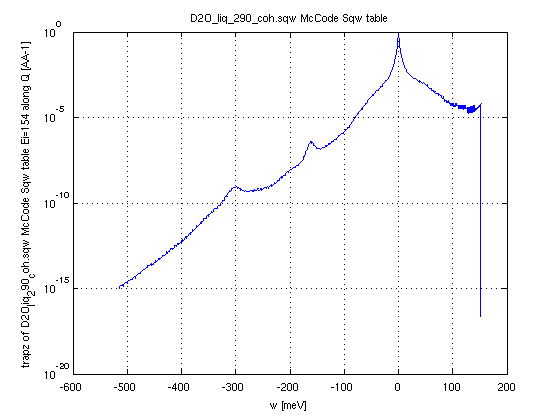In the following example, we use Molecular Dynamics data, symmetrize it, then apply the detailed balance to go from the 'classical' to the 'quantum' S(q,w). Finally, we extract the S(q,w) for a given incident energy Ei=154 meV and around 10 deg detector angle. And we plot the integral along momentum (axis 1) to show a spectrum.
• Sqw=iData_Sqw2D('D2O_liq_290_coh.sqw');
• SqwT = Bosify(Sqw_symmetrize(Sqw), 290);
• DDCS = dynamic_range(SqwT, 154, [5 15])
• plot(trapz(DDCS,2));
The energy axis must then be reversed and the incident energy be added, here 154 meV, to cope with the definition of the energy transfer used in nuclear engineering.

References for water data:

• O. K. Harling, “Slow Neutron Inelastic Scattering and the Dynamics of Heavy Water”,
Nucl. Sci. Eng., 33, 41 (1968). Provides heavy water data at Beta=1.5, Ei=38.6, meV T=299 K, and Ei=101 meV theta=60 deg. [link]
• F.G. Bischoff, M.L. Yeater, W.E. Moore, Nucl. Sci. Eng. 48 (1972) 266. Provides heavy water ToF data Ei=233 meV at θ=25 deg. [link]
• F.G. Bischoff et al., “Low Energy Neutron Inelastic Scattering”, RPI-328-87 (1967). Provides light water data Ei=154, 231, 631 meV at θ=60 deg.
• R. E. MacFarlane, LA-12639-MS (ENDF-356), 1994. [link]. Indicates light water rotational vibrations at 205 and 408 meV
• E. Liu (RPI) <http://homepages.rpi.edu/~danony/Papers/RND2011/ND_Sym_Liu_4_27_11.pdf> presents light water data at Ei=600 and Ei=154 meV, and compares to older data from Bischoff and Esch.
• J. I. Márquez Damian et al, Annals of Nuclear Energy 65 (2014) 280–289. [link] Gathers most of this data.
• Esch et al, Nuclear Science and Engineering: 46, 223-235, 1971. Provides light water data Ei=632 meV and 154 meV theta=10 14 25 40 60 150 deg. [link]

## Neutron diffraction

The iFit package includes a few features relevant to diffraction.

### Creating structure data files for McStas

The cif2hkl tool is derived from the CrysFML library (Juan Rodriguez-Carvajal and Javier Gonzalez-Platas). Its mai purpose is to read/convert material structure data files into HKL F2 lists, mainly for use with McStas.

• CIF
• FullProf PCR/CFL
• ShelX SHX/INS/RES

The generated file can be tuned for powders or single-crystal diffraction.

The syntax from iFit is:

output = cif2hkl('input', 'output', wavelength, 'mode')

where:

• input: data file to read/convert (CIF, PCR, CFL, SHX, INS, RES). It can also be given as a chemical formula with syntax 'cod: formula'.
• output: name/path of file for output. If not given or empty, an extension is added to the input file.
• wavelength: the wavelength used for generation of HKL peaks, in Angs. Default is 0.5 Angs. This affects the maximal reachable HKL indices.
• mode: conversion mode 'powder' or 'p'=powder, 'xtal' or 'x'=single crystal

The generated file name is returned after completion. It can e.g. be used with McStas components PowderN, Single_crystal, Isotropic_Sqw.

The input material structure can also be entered as a chemical formula in Hill notation (C, H, other atoms in alpha order) with syntax 'cod: formula' (access crystallography,net, requires a valid internet connexion).

NOTE: This requires proxy settings to be set (when behind a firewall)

• ProxyHost='proxy.ill.fr'; % Proxy address if you are behind a proxy [e.g. myproxy.mycompany.com or empty]
• ProxyPort=8888;           % Proxy port if you are behind a proxy [8888 or 0 or empty]
• java.lang.System.setProperty('http.proxyHost', ProxyHost);
• com.mathworks.mlwidgets.html.HTMLPrefs.setUseProxy(true);
• com.mathworks.mlwidgets.html.HTMLPrefs.setProxyHost(ProxyHost);
• java.lang.System.setProperty('http.proxyPort', num2str(ProxyPort));
• com.mathworks.mlwidgets.html.HTMLPrefs.setProxyPort(num2str(ProxyPort));

Examples:

• lmo = cif2hkl('LaMnO3.cif')
• lmo = cif2hkl('cod: La Mn O3')

### Pure Monte-Carlo Rietveld refinement

A Rietveld structure refinement model has been created. It is documented in Models Rietveld. It is very slow compared to usual analytical procedures such as FullProf, but it is based on a full McStas instrument model and goes much beyond the Caglioti formalism.

## Neutron Triple-Axis Spectrometers

The ResLibCal application allows to model a conventional neutron scattering Triple-Axis Spectrometer (TAS). It also allows to convolve a 4D S(q,w) dynamic structure factor model with the resolution function and perform fits. Read more in the dedicated miFit example for a phonon data set.

## Neutron Scattering Instruments with McStas

It is possible to control McStas instrument models from within iFit. By doing so, you will be able to perform parameter scans, optimisations, display the instrument code as well as its geometry, and mode. Read more at the McStas page.

It is also possible to export 2D and 4D S(q,w) Models into files for the S(q,w) McStas components Isotropic_Sqw and Single_crystal_inelastic. Refer to the dedicated section in the McStas page.

E. Farhi - iFit/iData loading data - Nov. 27, 2018 2.0.2 - back to Main iFit Page